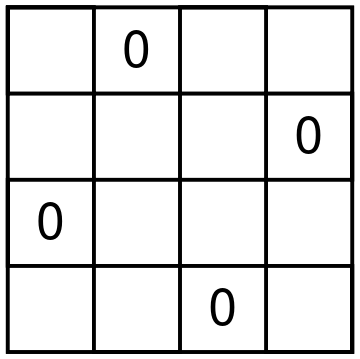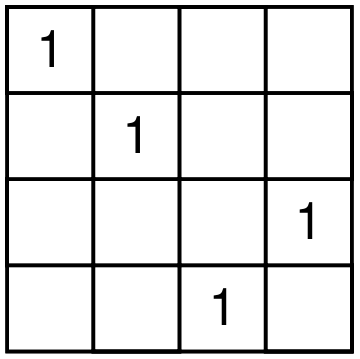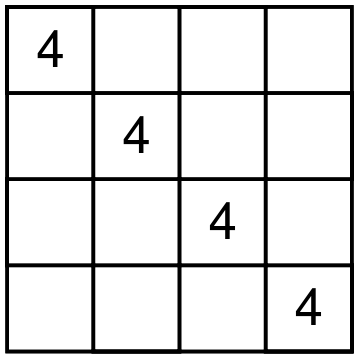## Shapes of Symbols in Latin Squares

Once John Conway showed me a cute way to enumerate Latin squares of size 4, up to the movements of the plane. It was a joint result with Alex Ryba, which is now written in a paper Kenning KenKen.

For starters, I want to remind you that a Latin square of size n is an n by n table filled with integers 1 through n, so that every row and column doesn’t have repeated integers. KenKen is a game that John Conway likes, where you need to recover a Latin square given some information about it.

Let me start by describing a particular shape of four cells that one digit can occupy in a Latin square of size 4. There are only seven different shapes. To get to the beautiful result, we need to number these seven shapes in a particular order starting from zero. The shapes are shown below.There are 12 different Latin squares up to movements of the square and relabeling the digits. Here is how Conway and Ryba matches shapes and squares. For each Latin square, take the shapes of all four digits, remove the duplicate shape numbers and sum the leftover shape numbers. You will get a unique number from 1 to 12 that represents a particular Latin square. For example, consider the square on the picture below.Digit 1 is shape 4, digits 2 and 4 form shape 2, and digit 3 forms shape 6. Shape 2 is used twice, and we ignore multiplicities. So we have shapes 2, 4, and 6 used. The resulting Latin square is number 2 + 4 + 6, that is 12. It is a fun exercise to try to find all the squares. For example, square 1 can only use shapes 0 and 1. But shape 1 uses exactly one corner. So the first square should use each of the digits in shape 1.

John likes finding interesting ways to remember which shape is which. You can find his and Alex’s suggestions in the paper which Alex submitted to the arxiv.

Oops! While I was writing this essay, arxiv rejected the paper.

Share: